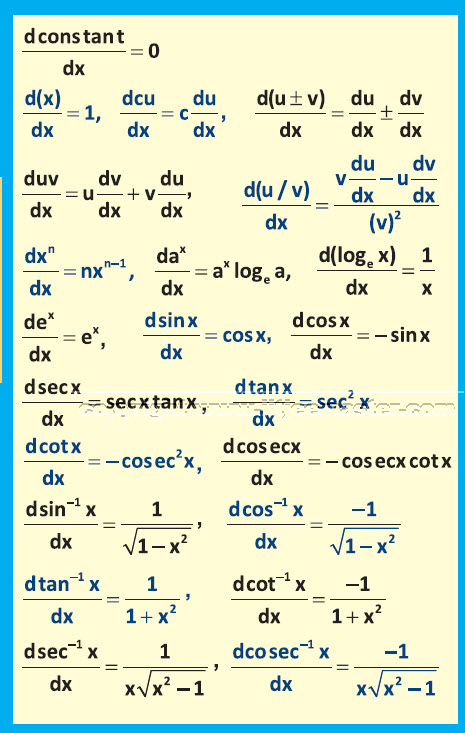In this maths video lecture for jee mains specially designed for IIT-JEE mains advance 2018 aspirants, students will learn about the topic differentiation. This topic was first devised by the famous mathematician, Sir Isaac Newton. Apart from this differential calculus, integrals etc are also his famous contributions.

Differentiation can be basically called as the process of finding a derivative. If f(x) is continuous and defined over an interval [a,b] and c belongs to [a,b]. Then the derivative of a function at x=c will be denoted by f ′ (c).

The first principle or ab-initio method of finding differential calculus is the most important in this video lecture. These JEE mathematics video lectures on differentiation provide you formulas and respective illustrations also. Always remember to practice as many as practical examples on the basis of the concepts given. This will always help you to remember the concepts clearly.

Now suppose with the help of first principle, we need to find the following: y= sinx , y+dy = sin (x+dx). In this case the value of dy = sin (x+dx) – sinx. In case need to find the differential calculus, the value will be dy/dx = limit dx tending to 0, [sin ( x+dx )n – sin x ]/ dx. In this concept you have to remember what is to substituted and where. You will be ever to find the complete illustration by watching the complete JEE differentiation video lecture.

With the help of this mathematics video lecture, you will also be able to find out the rate of change in y with respect to x expresses in terms of a ratio. In this way you can find the same for any function.

Differentiation is also one of the simple topics that helps you in scoring marks in your maths examination. However, throughout the video lecture, you need to maintain the flow of understanding and practice the illustrations after learning the concepts.

These video lectures on IIT-JEE mathematics series will always serve as a value-add to all your learning materials. Make sure to follow every video one after the other and prepare notes in case possible.

Differentiation as a JEE maths topic is crucial for all the students to learn and score maximum possible marks.